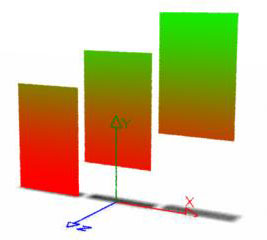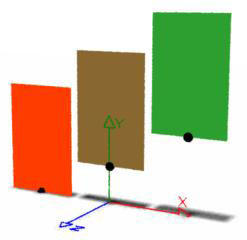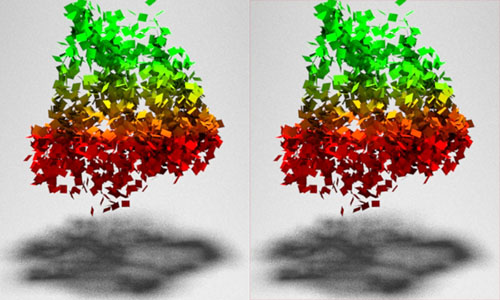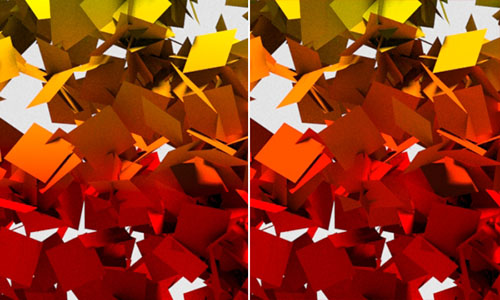### OSLColor By Height

#### Introduction

This tutorial addresses some issues relating to the shading of surfaces based on their position in world-space, in particular, their height above the origin of the world coordinate system. For example, a shader may be required to tint the leaves of a tree based on their height above the ground. A model of a tree might consist of several hundreds of leaves, consequently, it would be beneficial to have a single instance of a shader control the shading of the entire canopy of leaves.

Figures 1 and 2 show three polygons distributed along the x-axis of world-space. The local origin of each polygon is marked by small black spheres.Figure 1 Vertical color ramp, refer to shader listing 1Figure 2 Uniform shading, refer to shader listing 2

 Applying coloration based on the height of each shading point (`P`) of the geometry will produce vertical color ramp that may be undesireable. However, as shown in figures 3a and 4a when the polygons representing the leaves are small the color ramps are less noticeable. Using the height of the origin of each polygon to set their color, figures 3b and 4b, assigns each polygon a uniform color.Figures 3a and 3b colorByP.osl & colorByObjOrigin.oslFigures 4a and 4b colorByP.osl & colorByObjOrigin.osl

#### Coloration by Shading Point (P) Height

Listing 1 demonstrates how a shader's output color (`resultRGB`) is set according to the height of each surface point. The code creates a copy of `P` transformed to "world" space, the 'y' component of which is obtained by direct indexing,
`p`

Listing 1 - colorByP.osl

 ```shader colorByP(float minheight = 1.25, float maxheight = 2.5, color mincolor = color(1,0,0), color maxcolor = color(0,1,0), output color resultRGB = 0) { point p = transform("world", P); float blend = smoothstep(minheight, maxheight, p); resultRGB = mix(mincolor, maxcolor, blend); }```

For convenience, the shader uses the `smoothstep()` function to obtain a normalized value suitable for mixing colors.

#### Coloration by Surface Origin

Listing 2 demonstrates how to obtain the height of the local origin of a surface above the origin of the world coordinate system.

Listing 2 - colorByObjOrigin.osl

 ```shader colorByObjOrigin(float minheight = 1.25, float maxheight = 2.5, color mincolor = color(1,0,0), color maxcolor = color(0,1,0), output color resultRGB = 0) { point localOrigin = transform("object", "world", point(0,0,0)); float height = localOrigin; float blend = smoothstep(minheight, maxheight, height); resultRGB = mix(mincolor, maxcolor, blend); } ```

#### Coloration by User Attribute

Listing 3 demonstrates how a "user" attribute called "position" can be used set the surface color. The value of the attribute represents initial position of a surface and as such ensures the color of each "leaf" does not change during an animation - figure 5.

 ```AttributeBegin Attribute "user" "point position" [-0.847 1.061 0.578] Translate -0.847 1.501 0.578 Rotate -88.175 0.449 0.652 0.789 Rotate 880.333 0 0 1 Polygon "P" [-0.0625 0 -0.125 0.0625 0 -0.125 0.0625 0 0 -0.0625 0 0] "st" [0 0 0 1 1 1 1 0] AttributeEnd```

Listing 3 - colorByAttribute.osl

 ```shader colorByAttribute(float minheight = -1.0, float maxheight = 1.5, float offset = 0, color mincolor = color(1,0,0), color maxcolor = color(0,1,0), output color resultRGB = 0) { point pos; if(getattribute("user:position", pos) == 1) { float ht = offset + pos; float blend = smoothstep(minheight, maxheight, ht); resultRGB = mix(mincolor, maxcolor, blend); } } ```